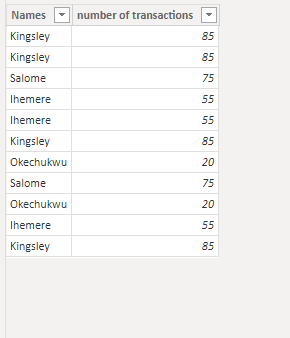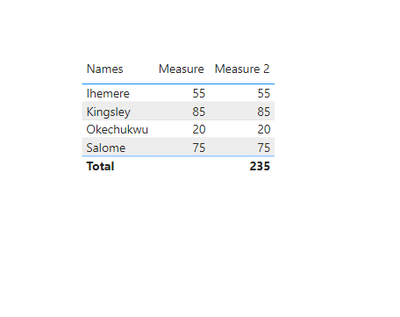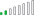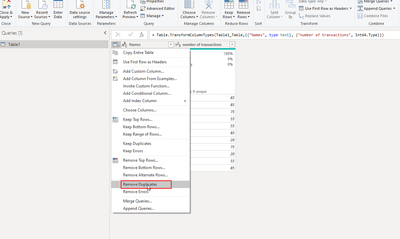cancel
Showing results for
Did you mean:Frequent Visitor

## row values not showing the correct result while total shows the correct value

Please I have a problem, first I have a table with repeated values like this

 Names number of transactions Kingsley 85 Kingsley 85 Salome 75 Ihemere 55 Ihemere 55 Kingsley 85 Okechukwu 20 Salome 75 Okechukwu 20 Ihemere 55 Kingsley 85

I want to get the sum of transactions considering that there are repeated record. There dupplicates are the same and outcome of a json column.

I used the following DAX functions to get the sum which seem correct.

max_tansaction = MAX('data'[number of transactions])
then this
total transaction = SUMX(DISTINCT('data'[Name]), [max_transaction])

when I visualized the data using table or matrix in power bi here is what I have

 Name number of transactions Kingsley 233 Okechukwu 224 Ihemere 232 Salome 231 Total 235

The total is correct but the values are not adding up.

Thamk you

8 REPLIES 8Frequent Visitor

I want to say thank you so much everyone for your support and I also very sorry, the problem was from me,

I was basing my calculations on the dimension table.

I had to do it from the fact table and I am good now.Community Support

Hi @dekings ,

Plaease try below steps:

1. below is my test table

Table:2. create measure with below dax formula

``````Measure =
VAR cur_name =
SELECTEDVALUE ( 'Table'[Names] )
VAR tmp =
FILTER ( ALL ( 'Table' ), 'Table'[Names] = cur_name )
VAR tmp1 =
CALCULATETABLE ( VALUES ( 'Table'[number of transactions] ), tmp )
VAR _a =
SUMX ( tmp1, [number of transactions] )
RETURN
_a
``````
``Measure 2 = SUMX(VALUES('Table'[Names]),[Measure])``

3. add a table visual with field and measurePlease refer the attached .pbix file.

Best regards,
Community Support Team_Binbin Yu
If this post helps, then please consider Accept it as the solution to help the other members find it more quickly.Community Champion

hi @dekings

try like:

measure =
SUMX(
VALUES(data[Name]),
"Sum",
CALCULATE(SUM(data[number of transactions]))
),
[Sum]
)Frequent Visitor

Thank you @FreemanZ  for your response,
the answer I got add all the rows but I need it to take only one value per name and add all the values such that when I visualize it I will get the second table in my questionSolution Sage

Hope the following helps: However, the best practice would be to remove duplicatesFrequent Visitor

So I tried using the dax you shared, it returned me to the answer I have getting.

No changes.Frequent Visitor

So this duplicate is not available in Power query level.
I created it using related function in dax from the dimension table.Resolver II

Hey @dekings ,

Why don't you remove the duplicated rows in the Power Query Editor? is it not possible for you?Cheers, LQ